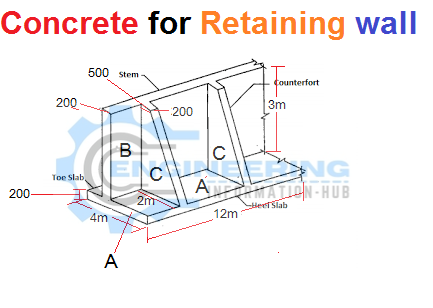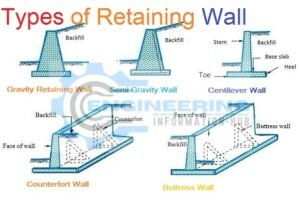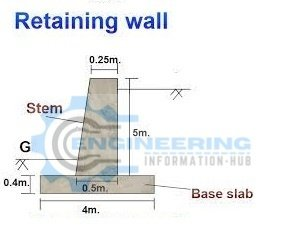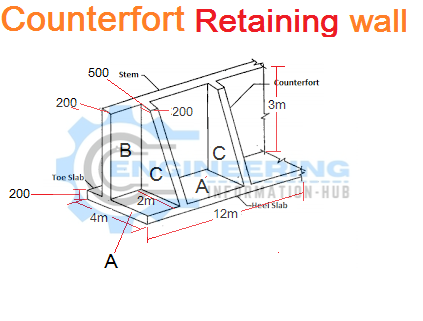# How To Calculate The Concrete for Retaining Wall

## Calculate The Concrete for Retaining WallIn this article, I explain What is the Retaining wall, How to calculate the concrete volume of Retaining wall types of Retaining walls.

### What is the Retaining wall?

A Retaining wall structure designed and created to resist the lateral pressure of soil, once there is the specified modification in ground elevation that exceeds the angle of repose of the soil. Hold walls unit used for supporting thereforeil laterally so it’s going to be maintained at utterly completely different levels on the two sides. Hold walls unit structures designed to restrain soil to a slope that it’d not naturally keep to (typically a steep, near-vertical, or vertical slope). they are used to certain soils between a pair of utterly completely different elevations typically in areas of a chunk of ground possessing undesirable slopes or in areas where the landscape should be shaped severely and engineered for tons of specific functions like slope farming or route overpasses,

### Types of Retaining wallNow we calculate the volume of Concrete for the Retaining wall.

we have given data in sketchLength of base slab=4m

suppose

width of base slab= 2m

height of slab = 0.4m

the volume of concrete of base slab= l×w×h

volume of concrete of base slab=4×2×0.4=3.2m³

### Now the volume of Concrete of stem

The stem of shape is trapezoidal

base length of trapezoidal=0.50m

top length of trapezoidal =0.25m

concrete volume of stem= {(base +top /2) ×height} ×length

concrete volume of stem= {(0.5+0.25/2)×5}×2

concrete volume of stem={0.75/2×5}×2=3.75m³

Now have Counterfort Retaining Wall

we calculate the concrete volume of Counterfort wall

we have given data in sketchwe have 3 portions of this wall

A PART Base Slab

Length =12m

width =4m

Thickness of base slab =0.200m

volume of concrete of base slab=L×W×Tickness

volume of concrete of base slab=12×4×0.200=9.6m³

Now Part B stem

given data

length of stem 12m

width of stem=0.200m

height of stem= 3m

concrete volume of stem=L×W×H

concrete volume of stem=12×0.200×3=7.2m³

### What is the Difference Between Pre-tension and Post-Tension

Now part C of counterfort

the shape of counterfort is trapezoidal

base length=2m

top length=0.500m

height =3m

length of trapezoidal=0.200m

concrete volume of counterfort= {(base+top/2)×height}×length

concrete volume of counterfort={(2+0.500/2)×3}×0.200

concrete volume of counterfort={(2.5/2)×3}×0.200=0.75m³

We have 2 counterforts so 0.75×2=15.m³

THANKS

### Raja Numan

Hi, My name is Engr. Raja Numan author of Engineering Information Hub and I am a Civil Engineer by Profession and I've specialized in the field of Quantity Surveying, Land Surveying as QC Engineer in national and multinational companies of Pakistan & Saudi Arabia.